Time: 3 Hours                                                                                                     Max. Marks: 100

NOTE: There are 9 Questions in all.

·      Question 1 is compulsory and carries 20 marks. Answer to Q. 1. must be written in the space provided for it in the answer book supplied and nowhere else.

·      Out of the remaining EIGHT Questions answer any FIVE Questions. Each question carries 16 marks.

·      Any required data not explicitly given, may be suitably assumed and stated.

Q.1       Choose the correct or best alternative in the following:                                         (2x10)

a.       The name of all functions end with a

(A)  pair of parenthesis                        (B)  semicolon

(C)   braces                                          (D)  colon

b.      A float variable can store any variable within the range of

(A)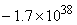to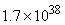(B)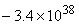to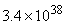(C)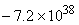to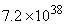(D)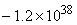to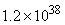c.   scanf() can be used for reading

(A)    double character                           (B)  single character

(C)  multiple characters                        (D)  no character

d.   ‘C’ allows a three-way transfer of control with the help of

(A)    unary operator                            (B) relational operator

(C)  ternary operator                           (D) comparison operator

e.   The statement that transfers control to the beginning of the loop is called

(A)     break statement                            (B)  exit statement

(C)  continue statement                        (D)  goto statement

f.    A variable which is visible only in the function in which it is defined, is called

(A)     static variable                               (B)  auto variable

(C)  external variable                           (D)  local variable

g.   The number of arguments supplied from the command line, by convention, is known as

(A)     arg c                                            (B)  arg v

(C)  #define                                         (D)  #include

h.   Output of the program given below is

int i;

main()

{

printf(“%d”, i);

}

(A)     1                                                 (B) 0

(C)  -1                                                (D) Null

i.    What will be the output of the following program?

main()

{

char *p = “ayqm”;

printf (“%c”, ++*(p++));

}

(A)   b                                                  (B) z

(C) q                                                   (D) n

j.    What will be the output of the following program?

main()

{

int i = 5;

printf(“%d”, i=++i==6);

}

(A)    0                                                  (B)  1

(C)  7                                                  (D)  6

Answer any FIVE Questions out of EIGHT Questions.

Each question carries 16 marks.

Q.2     a.   How problem definition phase plays an important role in terms of the problem-solving aspect?  Justify your answer.                                               (8)

b.   What is an algorithm? Write an algorithm to compute factorial of a number n where n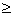0.               (8)

Q.3     a.   Write a program that reads a character from the keyboard and then prints it in the reverse case i.e. if the input is in upper case, the output will be in lower case and vice versa.                                 (8)

b.   Design an algorithm to evaluate the function sin(x) as defined by the infinite series expansion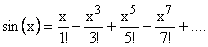(8)

Q.4     a.   Write short notes on following:

(i)                  Compilation errors.

b.   Write a program to read an integer number from the keyboard, add1 to it if the number read is even & again add 1 to it if the number is less than 20.  Otherwise, keep the number unchanged. (6)

c.   Give the difference between #include <stdio.h> and #include “stdio.h”.               (4)

Q.5     a.   Explain the use of default in switch block with the help of an example?                 (5)

b.   Write a program to read a set of real numbers & find the maximum & the minimum number entered with the help of do.. while loop.                        (6)

c.  Give the difference between break & continue statement.                                     (5)

Q.6     a.   Write a program to generate 100 random numbers lying in the range 0.0000 to 1.0000.  Evaluate their mean with the help of a function.                    (8)

b.   Define a function & explain why function prototype is essential.                           (8)

Q.7     a.   Write a program to sort an array of real numbers in ascending order.                   (8)

b.   Define the following:

(i)    Auto variables.                             (ii)  Static variables.

(iii)  External variables.                         (iv)  Register variables.                            (8)

Q.8     a.   What is a macro & how is it different from a pre processor?                               (6)

b.   Differentiate between #include Directive & # define Directive.                             (4)

c.   Write a program to read any number x & to evaluate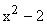.  Use a function to evaluate.  Call this function through its pointer.                  (6)

Q.9     a.   Explain in detail most commonly used dynamic memory allocation functions.                       (8)

b.   Write a program to create a link list of a set of integer numbers entered in the ascending order and add a new number to the linked list, such that the numbers in the new list also remain in the ascending order.    (8)